# Contest Animations with INTERP1

Today we're launching a new contest here on MATLAB Central: the MATLAB Flipbook Mini Hack. Why flipbook? It's an animation contest in which each animation gets exactly 48 frames, so it's like flipping through a 48-page pad of paper with slightly different drawings on each page. Check it out: entries are already pouring in!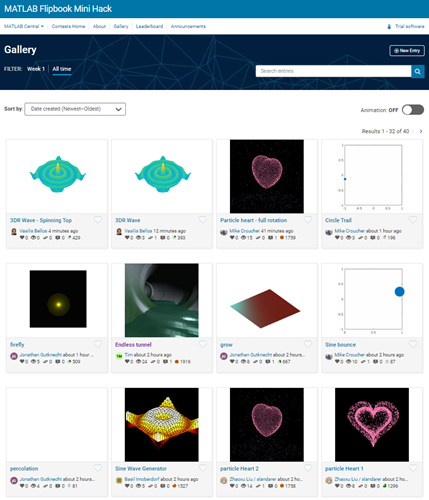In this post, I want to demonstrate some coding techniques that can make your animations easier. Suppose, for example, you wanted to animate a dot moving in a circle. Here the variable f is the frame number. It will take on the values from 1 to 48.
f = 1:48;
We want to use f to help us make an dot move in a circle. As f increments up to 48, we'll make the variable theta go from 0 to 2 pi. A perfect job for INTERP1! The INTERP1 function is a convenient way to do a linear map from one domain to another.
theta = interp1([0 48],[0 2*pi],f);
plot(f,theta,'.-',MarkerSize=12)
Let's pretty up this plot.
xlim([1 48])
ylim([0 2*pi])
set(gca,XTick=0:8:48)
set(gca,YTick=pi*(0:0.5:2),YTickLabel={"0","\pi/2","\pi","3\pi/2","2\pi"})
xlabel("Frame Number")
ylabel("Theta")
grid on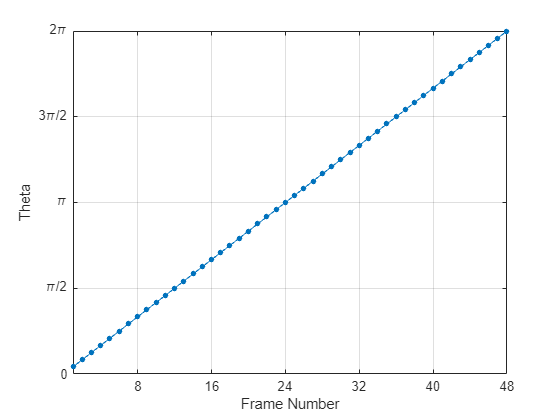This shows you how theta varies across all frames. It's all pretty straightforward, but I just love how INTERP1 washes away the hassle of working out the linear mapping.
Now it's time to build one frame of our animation. So instead of looking at all values of f, we'll just consider one. Appropriately, we'll start with frame 1.
f = 1;
Now we use our interpolation trick to map frame number into theta.
theta = interp1([0 48],[0 2*pi],f);
With theta defined, it's time to plot our moving dot. A little trig will put the dot in the right place.
plot(cos(theta),sin(theta),".", ...
MarkerSize=24)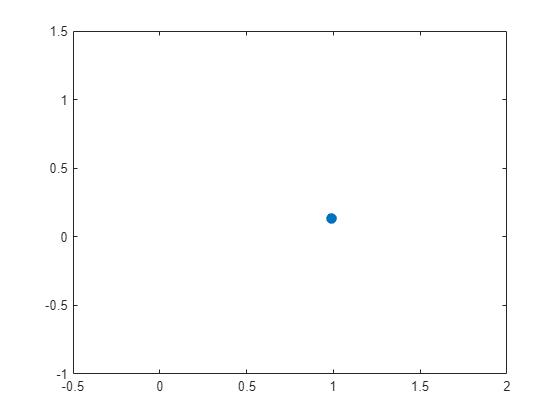Not much to look at so far! Let's make a circular race track that doesn't move so we can follow the action more clearly.
t = linspace(0,2*pi);
line(cos(t),sin(t))
And we'll square up the axis and reset the limits so the dot isn't bumping into the edges.
axis square
axis([-2 2 -2 2])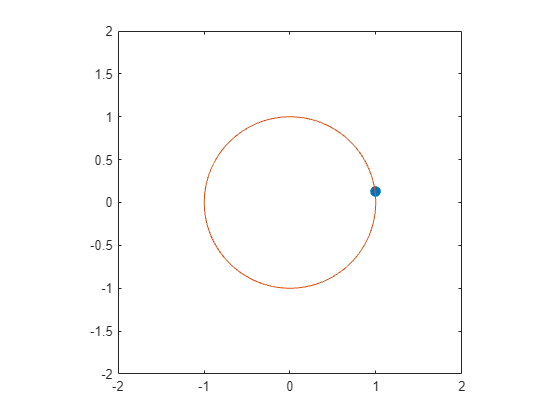Now we have the code that we put into the contest entry page. This code will create any of the frames 1 through 48 on demand. When you push the "Create Animation" button, the contest machinery does the rest of the magic. It generates all 48 frames in succession and stitches them into a single GIF image.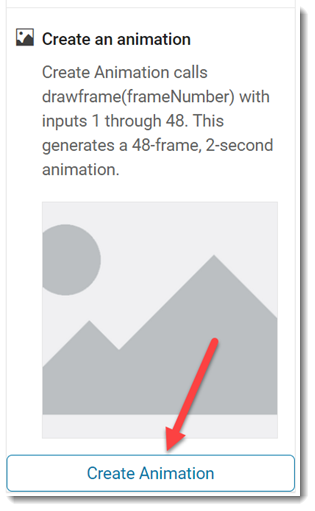Here is the result.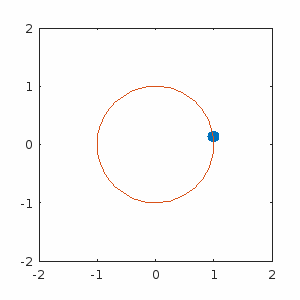There you have it: a two-second 48-frame movie masterpiece! I call it "Cutting a Hole in the Wall with a Blue Laser". Or "Blue Polar Bear Circles the North Pole". What would you call it?
That was a simple way to get started, but I feel we can do better. Let's do something more interesting with this circular motion.
First set up a landscape.
% Set up a landscape
peaks
z = 3*(1-x).^2.*exp(-(x.^2) - (y+1).^2) ...
- 10*(x/5 - x.^3 - y.^5).*exp(-x.^2-y.^2) ...
- 1/3*exp(-(x+1).^2 - y.^2)
material dull
axis vis3d off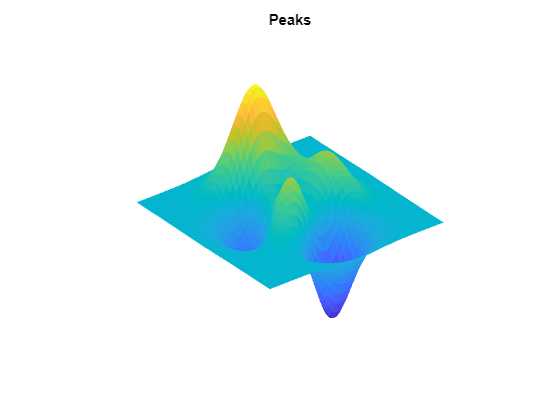Nice! Now we're going to create a light and move it around above this landscape. We can use our interpolation trick.
% Just showing frame 1 here
f = 1;
% Map onto theta
theta = interp1([0 48],[0 2*pi],f);
% Set up the light
ht = 4*sin(theta) + 4;
pos = [2*cos(theta) 2*sin(theta) ht];
light(Position=pos)
% The actual light is invisible, so we'll mark its position with a yellow dot
line(pos(1),pos(2),pos(3), ...
Marker=".",MarkerSize=24,Color="y")
set(gcf,Color=0.8*[1 1 1])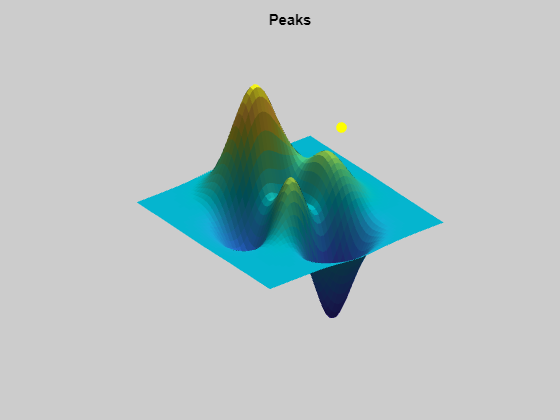Now as f changes, the light orbits around the landscape in three dimensions. Here's the final animation. Pretty cool, eh? It's certainly better than our first animation.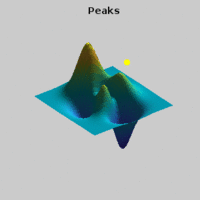I have found this contest to be a lot of fun, and very addictive. Maybe you will to. I encourage you to give it a try. Now that you know the INTERP1 trick, you have the secret to making your images dance.

|